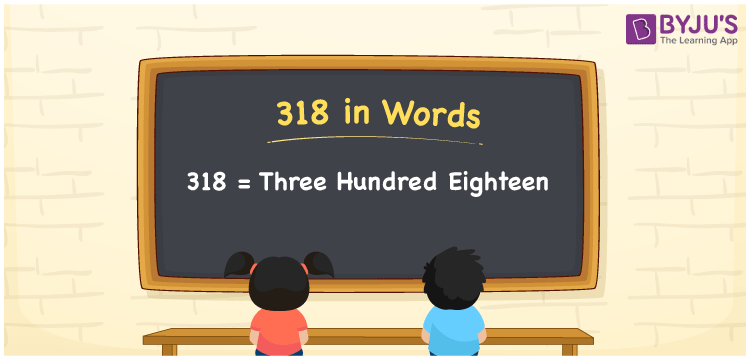# 318 in words

318 in words is written as Three Hundred and Eighteen. 318 represents the count or value. The article on Counting Numbers can give you an idea about count or counting. The number 318 is a 3 digit number that is used in expressions related to money, days, distance, length, weight and so on. Let us consider an example for 318. “I need to take the bus numbered Three Hundred and Eighteen to reach my school.” Another example is “At a wedding, the caterers needed Three Hundred and Eighteen kgs of ghee to prepare a special sweet dish”.

 318 in words Three Hundred and Eighteen Three Hundred and Eighteen in Numbers 318

## 318 in English Words## How to Write 318 in Words?

We can convert 318 to words using a place value chart. The number 318 has 3 digits, so let’s make a chart that shows the place value up to 3 digits.

 Hundreds Tens Ones 3 1 8

Thus, we can write the expanded form as:

3 × Hundred + 1 × Ten + 8 × One

= 3 × 100 + 1 × 10 + 8 × 1

= 318

= Three Hundred and Eighteen.

318 is the Natural number that is succeeded by 317 and preceded by 319.

318 in words – Three Hundred and Eighteen.

Is 318 an odd number? – No.

Is 318 an even number? – Yes.

Is 318 a perfect square number? – No.

Is 318 a perfect cube number? – No.

Is 318 a prime number? – No.

Is 318 a composite number? – Yes.

## Solved Example

1. Write the number 318 in expanded form

Solution: 3 × 100 + 1 × 10 + 8 × 1

We can write 318 = 300 + 10 + 8

= 3 × 100 + 1 × 10 + 8 × 1.

## Frequently Asked Questions on 318 in words

Q1

### How to write the number 318 in words?

318 in words is written as Three Hundred and Eighteen.
Q2

### State True or False. 318 is divisible by 3?

True. 318 is divisible by 3.
Q3

### Is 318 a perfect cube number?

No. 318 is not a perfect cube number.